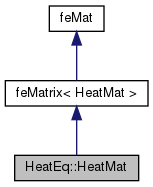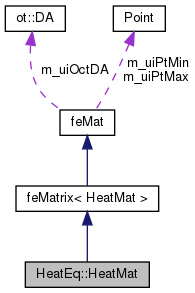Dendro  5.01 Dendro in Greek language means tree. The Dendro library is a large scale (262K cores on ORNL's Titan) distributed memory adaptive octree framework. The main goal of Dendro is to perform large scale multiphysics simulations efficeiently in mordern supercomputers. Dendro consists of efficient parallel data structures and algorithms to perform variational ( finite element) methods and finite difference mthods on 2:1 balanced arbitary adaptive octrees which enables the users to perform simulations raning from black holes (binary black hole mergers) to blood flow in human body, where applications ranging from relativity, astrophysics to biomedical engineering.
HeatEq::HeatMat Class Reference
Inheritance diagram for HeatEq::HeatMat:[legend]
Collaboration diagram for HeatEq::HeatMat:[legend]

## Public Member Functions

HeatMat (ot::DA *da, unsigned int dof=1)
: constructor

~HeatMat ()
default destructor

virtual void elementalMatVec (const VECType *in, VECType *out, double *coords=NULL, double scale=1.0)

bool preMatVec (const VECType *in, VECType *out, double scale=1.0)
things need to be performed before matvec (i.e. coords transform)

bool postMatVec (const VECType *in, VECType *out, double scale=1.0)
things need to be performed after matvec (i.e. coords transform)

double gridX_to_X (double x)
octree grid x to domin x

double gridY_to_Y (double y)
octree grid y to domin y

double gridZ_to_Z (double z)
octree grid z to domin z

int cgSolve (double *x, double *b, int max_iter, double &tol, unsigned int var=0)Public Member Functions inherited from feMatrix< HeatMat >
feMatrix (ot::DA *da, unsigned int dof=1)
constructs an FEM stiffness matrix class. More...

virtual void matVec (const VECType *in, VECType *out, double scale=1.0)
Computes the LHS of the weak formulation, normally the stifness matrix times a given vector. More...

HeatMat & asLeaf ()
static cast to the leaf node of the inheritance

bool preMatVec (const VECType *in, VECType *out, double scale=1.0)
executed just before the matVec loop in matvec function More...

bool postMatVec (const VECType *in, VECType *out, double scale=1.0)
executed just after the matVec loop in matvec function More...

bool preMat ()
executed before the matrix assembly

bool postMat ()
executed after the matrix assembly

void getElementalMatrix (unsigned int eleID, std::vector< ot::MatRecord > &records, double *coords)
Compute the elemental Matrix. More...Public Member Functions inherited from feMat
feMat (ot::DA *da)
: feMat constructor More...

~feMat ()
deconstructor

void setProblemDimensions (const Point &pt_min, const Point &pt_max)
set the problem dimensionProtected Attributes inherited from feMatrix< HeatMat >
unsigned int m_uiDof
number of dof

VECType * m_uiEleVecIn
element nodal vec in

VECType * m_uiEleVecOut

double * m_uiEleCoordsProtected Attributes inherited from feMat
ot::DAm_uiOctDA
: pointer to OCT DA

ot::DAType m_uiDaType
: type of the DA

Point m_uiPtMin
problem domain min point

Point m_uiPtMax
problem domain max point

## ◆ elementalMatVec()

 void HeatEq::HeatMat::elementalMatVec ( const VECType * in, VECType * out, double * coords = `NULL`, double scale = `1.0` )
virtual

elemental matvec

Implements feMatrix< HeatMat >.

The documentation for this class was generated from the following files:
• FEM/examples/include/heatMat.h
• FEM/examples/src/heatMat.cpp